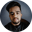Related Tags

lodash
communitycreator

# How to get a random element from an array using LodashShubham Singh Kshatriya

### Overview

In Lodash, we can get a random element from an array using the following methods:

• _.sample() method
• _.sampleSize() method

### Syntax

_.sample(array);
Syntax of _.clone()

### Parameters

This method accepts the following parameters:

• array: This is the array from which a random element is retrieved.

### Return value

This method returns a random element from the array.

### Example

Let’s look at an example of getting a random element from an array using the _.sample() method in the code snippet below.

Console

### Explanation

In the HTML tab, we see the following:

• Line 5: We import the lodash script.

In the JavaScript tab, we see the following:

• Line 2: We create an array and initialize it.
• Line 5: We get a random element from the array using the _.sample() method.
• Line 8: We print the random element on the console.

### Syntax

_.sampleSize(array, n);
Syntax of _.sampleSize()

### Parameters

This method accepts the following parameters:

• array: This is the array from which n random elements are retrieved.
• n : This is the number of random elements to be retrieved.

### Return value

This method returns an array containing n random elements from the array.

### Example

Let’s look at an example of getting n random elements from an array using the _.sampleSize() method in the code snippet below.

Console

### Explanation

In the HTML tab, we see the following:

• Line 5: We import the lodash script.

In the JavaScript tab, we see the following:

• Line 2: We create an array and initialize it.
• Line 5: We create a variable n and initialize it.
• Line 8: We get n random elements from the array using the _.sampleSize() method.
• Line 11: We print the random element on the console.

RELATED TAGS

lodash
communitycreator

CONTRIBUTORShubham Singh Kshatriya
RELATED COURSES

View all Courses

Keep Exploring

Learn in-demand tech skills in half the time